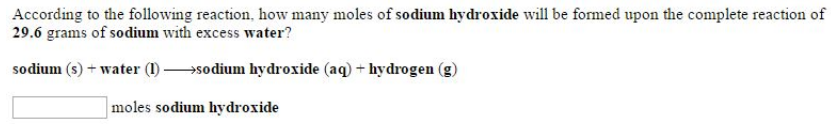# Problem: According to the following reaction, how many moles of sodium hydroxide will be formed upon the complete reaction of 29.6 grams of sodium with excess water? sodium (s) + water (l) → sodium hydroxide (aq) + hydrogen (g)

🤓 Based on our data, we think this question is relevant for Professor DeSimone's class at UA.

###### FREE Expert Solution###### Problem Details

According to the following reaction, how many moles of sodium hydroxide will be formed upon the complete reaction of 29.6 grams of sodium with excess water?

sodium (s) + water (l) → sodium hydroxide (aq) + hydrogen (g)